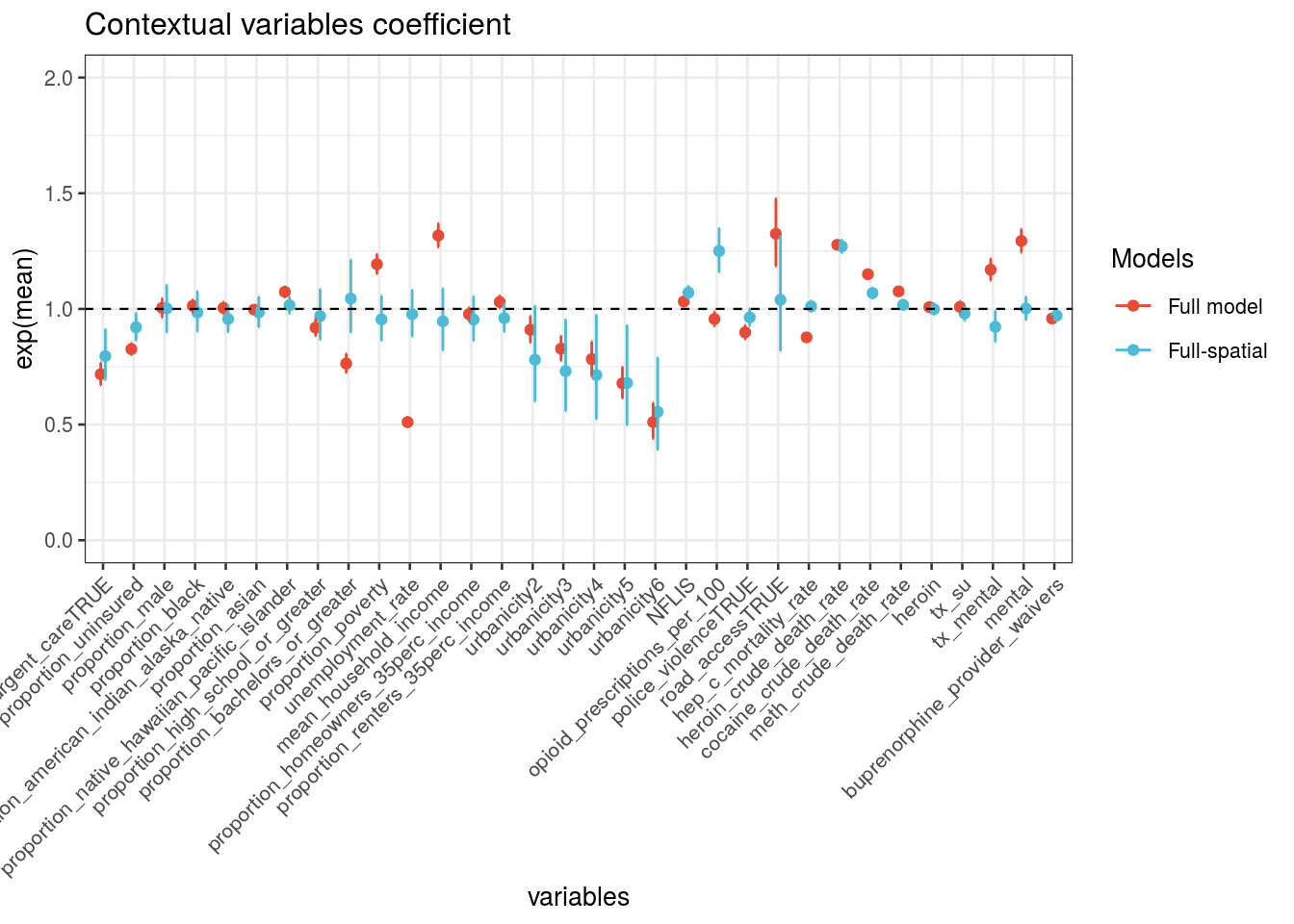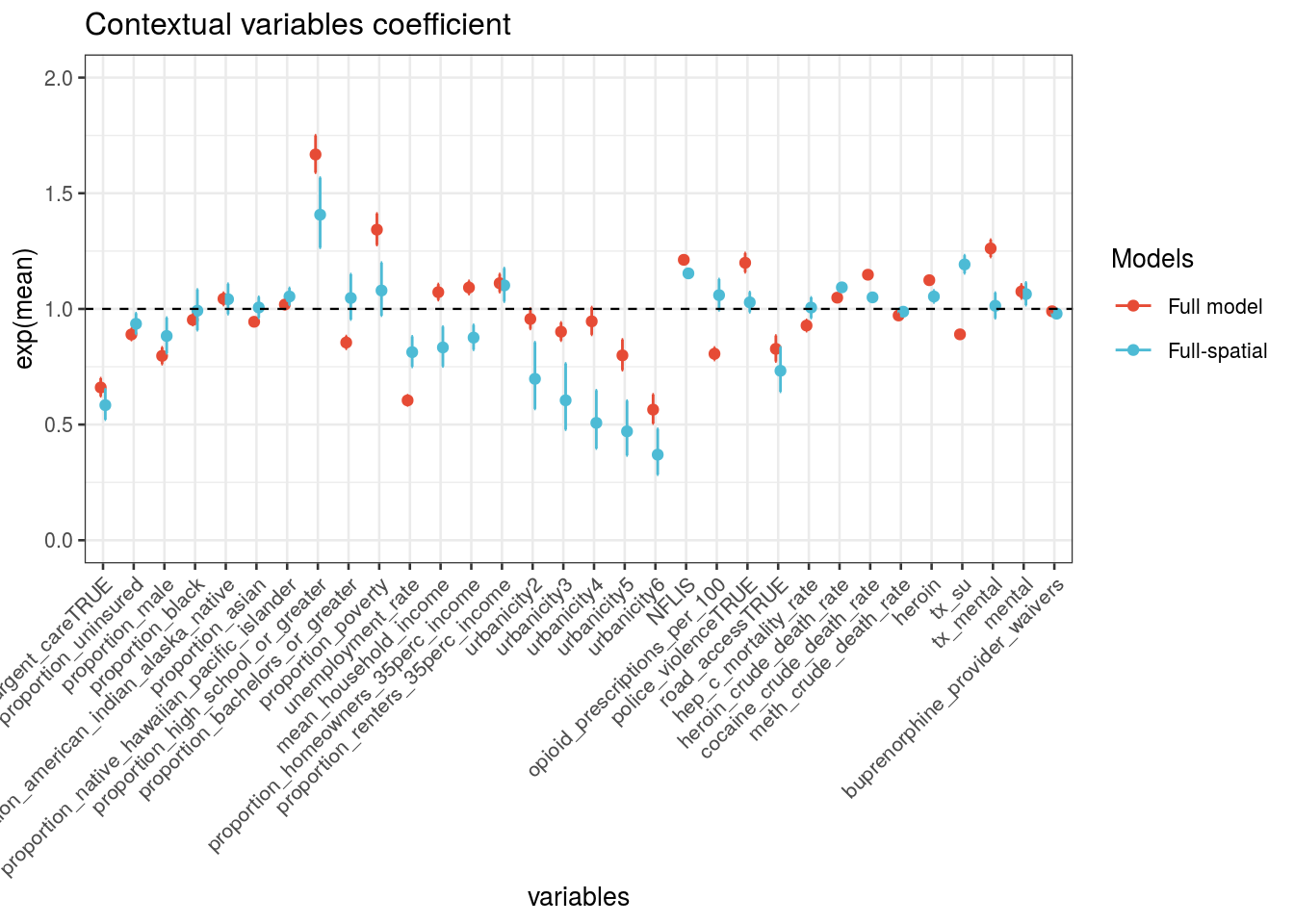# Chapter 4 Covars

## 4.1 LIBRARIES

``````library(tidyverse); library(epiDisplay); library(lubridate)
library(spdep); library(sf); library(sp)
library(maptools); library(rgdal); library(INLA);
library(MatrixModels); library(graph); library(Rgraphviz)``````

## 4.2 ADMINISTRATIVE AND SPATIAL DATA

``````data <- read.csv("./_data/usable_data_binary_outcomes_2013-17_previous_year_corrected.csv", stringsAsFactors = F)

usa.sf <- st_read("./_data/GIS/tl_2017_us_county/tl_2017_us_county.shp",  stringsAsFactors = F) %>%
#filter(STATEFP=="01") %>%
filter(STATEFP!="02", STATEFP!="15", STATEFP!="60", STATEFP!="66", STATEFP!="69", STATEFP!="72", STATEFP!="78") %>%
mutate(County.Code = as.numeric(GEOID))``````
``````## Reading layer `tl_2017_us_county' from data source `/research-home/gcarrasco/EMMERG_MAP2/_data/GIS/tl_2017_us_county/tl_2017_us_county.shp' using driver `ESRI Shapefile'
## Simple feature collection with 3233 features and 17 fields
## geometry type:  MULTIPOLYGON
## dimension:      XY
## bbox:           xmin: -179.2311 ymin: -14.60181 xmax: 179.8597 ymax: 71.43979
## epsg (SRID):    4269
``````index <- usa.sf %>%
dplyr::select(County.Code) %>%
st_set_geometry(NULL) %>%
mutate(index = 1:nrow(usa.sf),
index2 = index)

dat <- data %>%
left_join(index, by="County.Code") %>%
mutate(fenta = as.numeric(any_fentanyl))

n<-nrow(dat)``````

## 4.3 MODEL SPECIFICATIONS

``````# Create adjacency matrix
nb.map <- poly2nb(usa.sf)
nb2INLA("map.graph",nb.map)

# FORMULAS
#block1
pv_2<-c(#formula = fenta ~ 1 + f(index, model = "bym", graph = "map.graph") + f(Year, model = "rw1") + log_average_pill_count_per_100000,
formula = fenta ~ 1 + f(index, model = "bym", graph = "map.graph") + f(Year, model = "rw1") + factor(urbanicity),
formula = fenta ~ 1 + f(index, model = "bym", graph = "map.graph") + f(Year, model = "rw1") + unemployment,
formula = fenta ~ 1 + f(index, model = "bym", graph = "map.graph") + f(Year, model = "rw1") + median_income,
formula = fenta ~ 1 + f(index, model = "bym", graph = "map.graph") + f(Year, model = "rw1") + proportion_poverty,
formula = fenta ~ 1 + f(index, model = "bym", graph = "map.graph") + f(Year, model = "rw1") + proportion_uninsured,
formula = fenta ~ 1 + f(index, model = "bym", graph = "map.graph") + f(Year, model = "rw1") + homeowners_35perc_income
)

#block2
pv_2<-c(formula = fenta ~ 1 + f(index, model = "bym", graph = "map.graph") + f(Year, model = "rw1") + proportion_no_high_school,
formula = fenta ~ 1 + f(index, model = "bym", graph = "map.graph") + f(Year, model = "rw1") + proportion_white,
formula = fenta ~ 1 + f(index, model = "bym", graph = "map.graph") + f(Year, model = "rw1") + log_population_density,
formula = fenta ~ 1 + f(index, model = "bym", graph = "map.graph") + f(Year, model = "rw1") + previous_year_police_violence,
formula = fenta ~ 1 + f(index, model = "bym", graph = "map.graph") + f(Year, model = "rw1") + road_access,
formula = fenta ~ 1 + f(index, model = "bym", graph = "map.graph") + f(Year, model = "rw1") + urgent_care
)

#block3
pv_2<-c(formula = fenta ~ 1 + f(index, model = "bym", graph = "map.graph") + f(Year, model = "rw1") + median_income + proportion_no_high_school,
formula = fenta ~ 1 + f(index, model = "bym", graph = "map.graph") + f(Year, model = "rw1") + median_income + proportion_no_high_school + proportion_white,
formula = fenta ~ 1 + f(index, model = "bym", graph = "map.graph") + f(Year, model = "rw1") + median_income + proportion_no_high_school + proportion_white + homeowners_35perc_income,
formula = fenta ~ 1 + f(index, model = "bym", graph = "map.graph") + f(Year, model = "rw1") + median_income + proportion_no_high_school + proportion_white + homeowners_35perc_income + urgent_care,
formula = fenta ~ 1 + f(index, model = "bym", graph = "map.graph") + f(Year, model = "rw1") + median_income + proportion_no_high_school + proportion_white + homeowners_35perc_income + urgent_care + proportion_poverty
)

#block4
pv_2<-c(formula = fenta ~ 1 + f(index, model = "bym", graph = "map.graph") + f(Year, model = "rw1") + median_income + proportion_no_high_school + proportion_white + homeowners_35perc_income + urgent_care + proportion_poverty + proportion_uninsured,
formula = fenta ~ 1 + f(index, model = "bym", graph = "map.graph") + f(Year, model = "rw1") + median_income + proportion_no_high_school + proportion_white + homeowners_35perc_income + urgent_care + proportion_poverty + proportion_uninsured + previous_year_police_violence,
formula = fenta ~ 1 + f(index, model = "bym", graph = "map.graph") + f(Year, model = "rw1") + median_income + proportion_no_high_school + proportion_white + homeowners_35perc_income + urgent_care + proportion_poverty + proportion_uninsured + previous_year_police_violence + factor(urbanicity),
formula = fenta ~ 1 + f(index, model = "bym", graph = "map.graph") + f(Year, model = "rw1") + median_income + proportion_no_high_school + proportion_white + homeowners_35perc_income + urgent_care + proportion_poverty + proportion_uninsured + previous_year_police_violence + factor(urbanicity) + road_access,
formula = fenta ~ 1 + f(index, model = "bym", graph = "map.graph") + f(Year, model = "rw1") + median_income + proportion_no_high_school + proportion_white + homeowners_35perc_income + urgent_care + proportion_poverty + proportion_uninsured + previous_year_police_violence + factor(urbanicity) + road_access + unemployment
)

# Helper Functions
source("~/CLIM_MAL/CLIM_MAL_v4_functions.R")``````

## 4.4 Model Estimation

``````names(pv_2)<-pv_2

test_pv_2 <- pv_2 %>% purrr::map(~inla.batch.safe(formula = .))

pv_2.s <- test_pv_2 %>%
purrr::map(~Rsq.batch.safe(model = ., dic.null = null[]\$dic, n = n)) %>%
bind_rows(.id = "formula") %>% mutate(id = row_number())``````
``````##  0.3128521
##  0.2524835
##  0.3228761
##  0.3213586
##  0.321137
##  0.3215114
##  0.3220558
##  0.321909
##  0.3150553
##  0.2834885
##  0.3213674
##  0.3213674
##  0.2457204
##  0.3217414
##  0.320817
##  0.3216095
##  0.3207021
##  0.3220466
##  0.3187936
##  0.3152631
##  0.3184511
##  0.3151757``````
``````## # A tibble: 23 x 8
##    formula                     Rsq   DIC    pD `log score`  waic `waic pD`    id
##    <chr>                     <dbl> <dbl> <dbl>       <dbl> <dbl>     <dbl> <int>
##  1 "fenta ~ 1 + f(index, m…  0.313 2601.  554.      0.0669 2483.      366.     1
##  2 "fenta ~ 1 + f(index, m…  0.252 4241.  580.      0.108  4055.      352.     2
##  3 "fenta ~ 1 + f(index, m…  0.323  NaN   NaN       0.0660 2381.      420.     3
##  4 "fenta ~ 1 + f(index, m…  0.321  Inf   Inf       0.0681 2433.      420.     4
##  5 "fenta ~ 1 + f(index, m…  0.321  NaN   NaN       0.0681 2435.      418.     5
##  6 "fenta ~ 1 + f(index, m…  0.322  NaN   NaN       0.0679 2425.      418.     6
##  7 "fenta ~ 1 + f(index, m…  0.322  NaN   NaN       0.0686 2379.      412.     7
##  8 "fenta ~ 1 + f(index, m…  0.322  NaN   NaN       0.0674 2395.      413.     8
##  9 "fenta ~ 1 + f(index, m… NA       NA    NA      NA        NA        NA      9
## 10 "fenta ~ 1 + f(index, m…  0.315  Inf   Inf       0.0706 2571.      417.    10
## # … with 13 more rows``````
``pv_2.s %>% plot_score()``## 4.5 Output

### 4.5.1 Year Random Effects

``test_pv_2[] %>% plot_random2(y_lab = "Odd Ratio")````summary(test_pv_2[])``
``````##
## Call:
##    c("inla(formula = formula, family = \"binomial\", data = dat1, verbose
##    = F, ", " control.compute = list(config = T, dic = T, cpo = T, waic =
##    T), ", " control.predictor = list(link = 1, compute = TRUE),
##    control.fixed = list(correlation.matrix = T))" )
## Time used:
##     Pre = 18.7, Running = 228, Post = 56.3, Total = 303
## Fixed effects:
##                             mean    sd 0.025quant 0.5quant 0.975quant   mode
## (Intercept)               -5.398 0.743     -6.928   -5.374     -4.010 -5.327
## median_income              0.000 0.000      0.000    0.000      0.000  0.000
## proportion_no_high_school -0.137 0.029     -0.195   -0.136     -0.081 -0.135
## proportion_white          -0.084 0.008     -0.101   -0.084     -0.068 -0.084
## homeowners_35perc_income   0.030 0.022     -0.014    0.030      0.073  0.030
## urgent_careTRUE           -4.662 0.355     -5.385   -4.651     -3.999 -4.626
##                           kld
## (Intercept)                 0
## median_income               0
## proportion_no_high_school   0
## proportion_white            0
## homeowners_35perc_income    0
## urgent_careTRUE             0
##
## Linear combinations (derived):
##                           ID   mean    sd 0.025quant 0.5quant 0.975quant   mode
## (Intercept)                1 -5.398 0.743     -6.872   -5.392     -3.955 -5.380
## median_income              2  0.000 0.000      0.000    0.000      0.000  0.000
## proportion_no_high_school  3 -0.137 0.029     -0.195   -0.136     -0.080 -0.136
## proportion_white           4 -0.084 0.008     -0.101   -0.084     -0.068 -0.084
## homeowners_35perc_income   5  0.030 0.022     -0.014    0.030      0.073  0.030
## urgent_careTRUE            6 -4.662 0.355     -5.379   -4.654     -3.991 -4.633
##                           kld
## (Intercept)                 0
## median_income               0
## proportion_no_high_school   0
## proportion_white            0
## homeowners_35perc_income    0
## urgent_careTRUE             0
##
## Random effects:
##   Name     Model
##     index BYM model
##    Year RW1 model
##
## Model hyperparameters:
##                                          mean    sd 0.025quant 0.5quant
## Precision for index (iid component)     0.541 0.258      0.242    0.475
## Precision for index (spatial component) 0.035 0.006      0.024    0.035
## Precision for Year                      0.068 0.047      0.013    0.056
##                                         0.975quant  mode
## Precision for index (iid component)          1.213 0.376
## Precision for index (spatial component)      0.048 0.034
## Precision for Year                           0.187 0.036
##
## Expected number of effective parameters(stdev): 762.53(16.54)
## Number of equivalent replicates : 24.73
##
## Watanabe-Akaike information criterion (WAIC) ...: 2280.26
## Effective number of parameters .................: 382.10
##
## Marginal log-Likelihood:  -1275.29
## CPO and PIT are computed
##
## Posterior marginals for the linear predictor and
##  the fitted values are computed``````

### 4.5.2 Spatial Random Effects

``test_pv_2[] %>% plot_random_map2(map = usa.sf, name= "model-17", id = County.Code, col1 = NA)``### 4.5.3 RMSE

``````# Spatial vs. (spatio-temporal / Covars)
plot_rmse(dat1 = dat, var = fenta, lab1 = c("Spatio-temporal", "Covars"), col1 = NA,
baseline = spatial[], model1 = spatial[], model2 = test_pv_2[],
map = usa.sf, id = County.Code)``````